In this tutorial we will cover how we can create a gradient style that will be used in various widgets. This tutorial will cover how to create a gradient image / style that can be applied to UI.

## Learning Objectives

• How to use `ImageWithProvider`` to create Image Widgets

• Create functions to interpolate between colors

• Apply custom styles to widgets

## Step 1: Add the Extension

### Step 1.1: Clone the repository

Clone the `gradient-tutorial-start` branch of the `kit-extension-sample-ui-window` GitHub repository:

```git clone -b gradient-tutorial-start https://github.com/NVIDIA-Omniverse/kit-extension-sample-ui-window.git
```

This repository contains the assets you use in this tutorial.

### Step 1.2: Open Extension Search Paths

Go to Extension Manager -> Gear Icon -> Extension Search Path: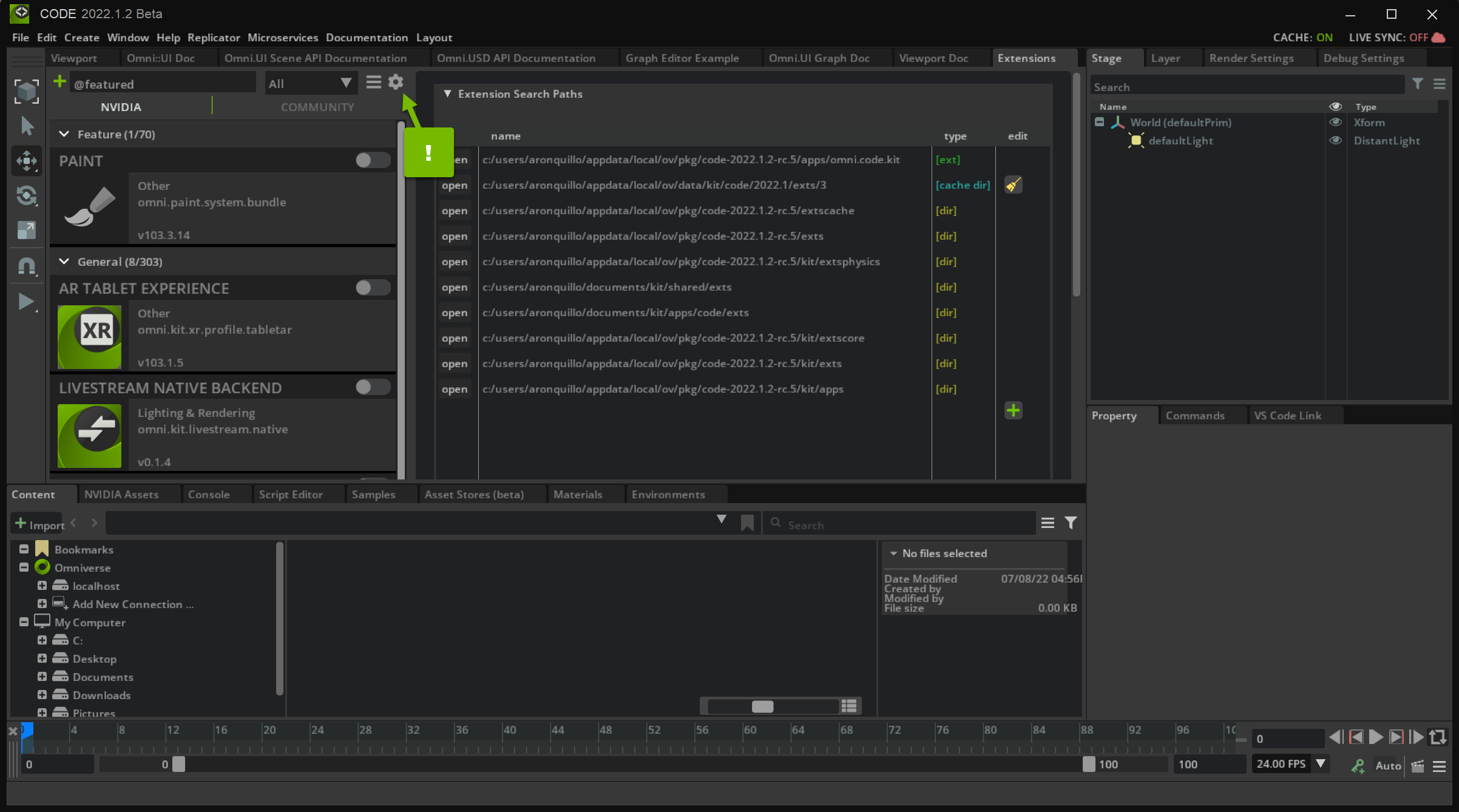### Step 1.3: Add the Path

Create a new search path to the exts directory of your Extension by clicking the green plus icon and double-clicking on the path field: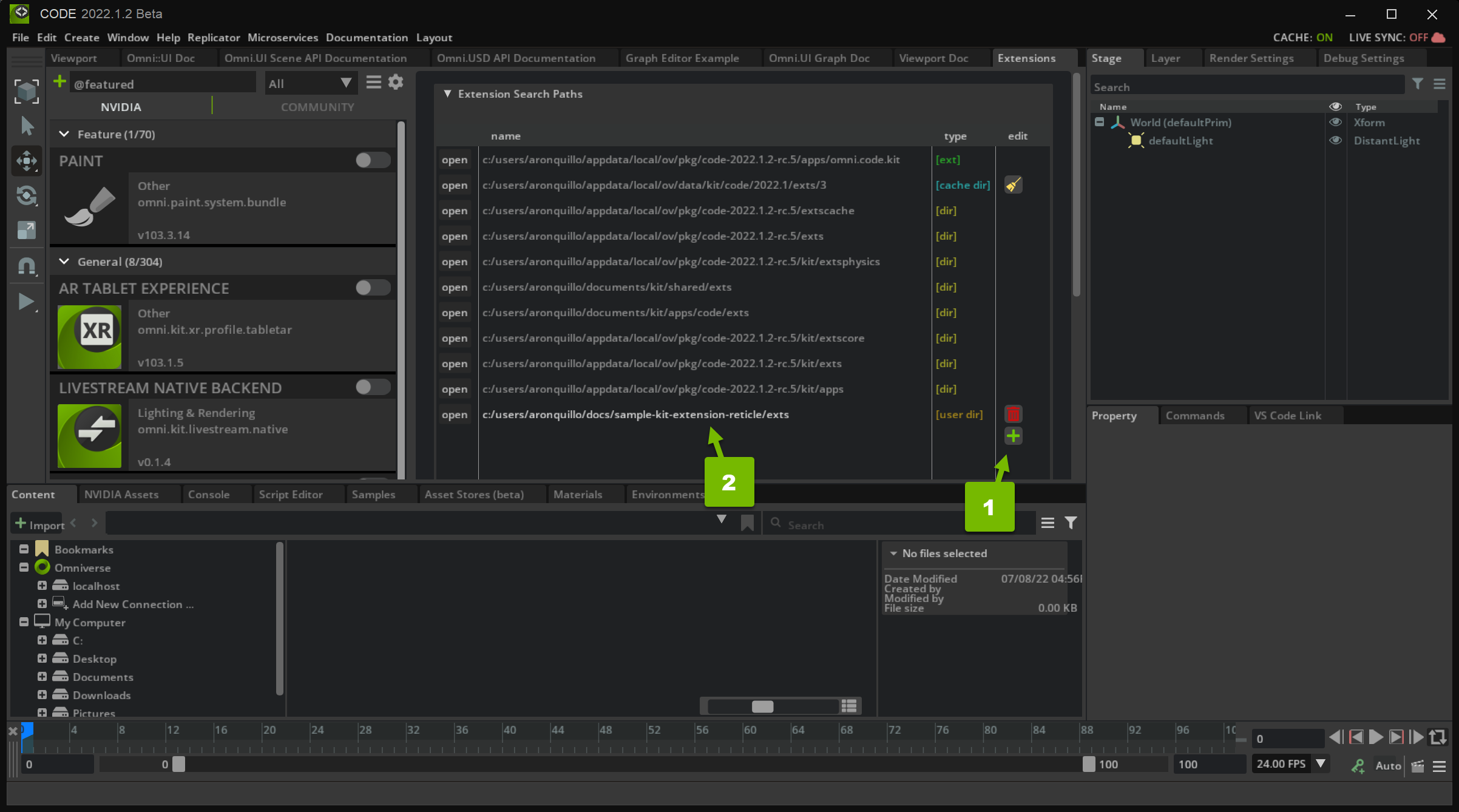When you submit your new search path, you should be able to find your extension in the Extensions list.

### Step 1.4: Enable the Extension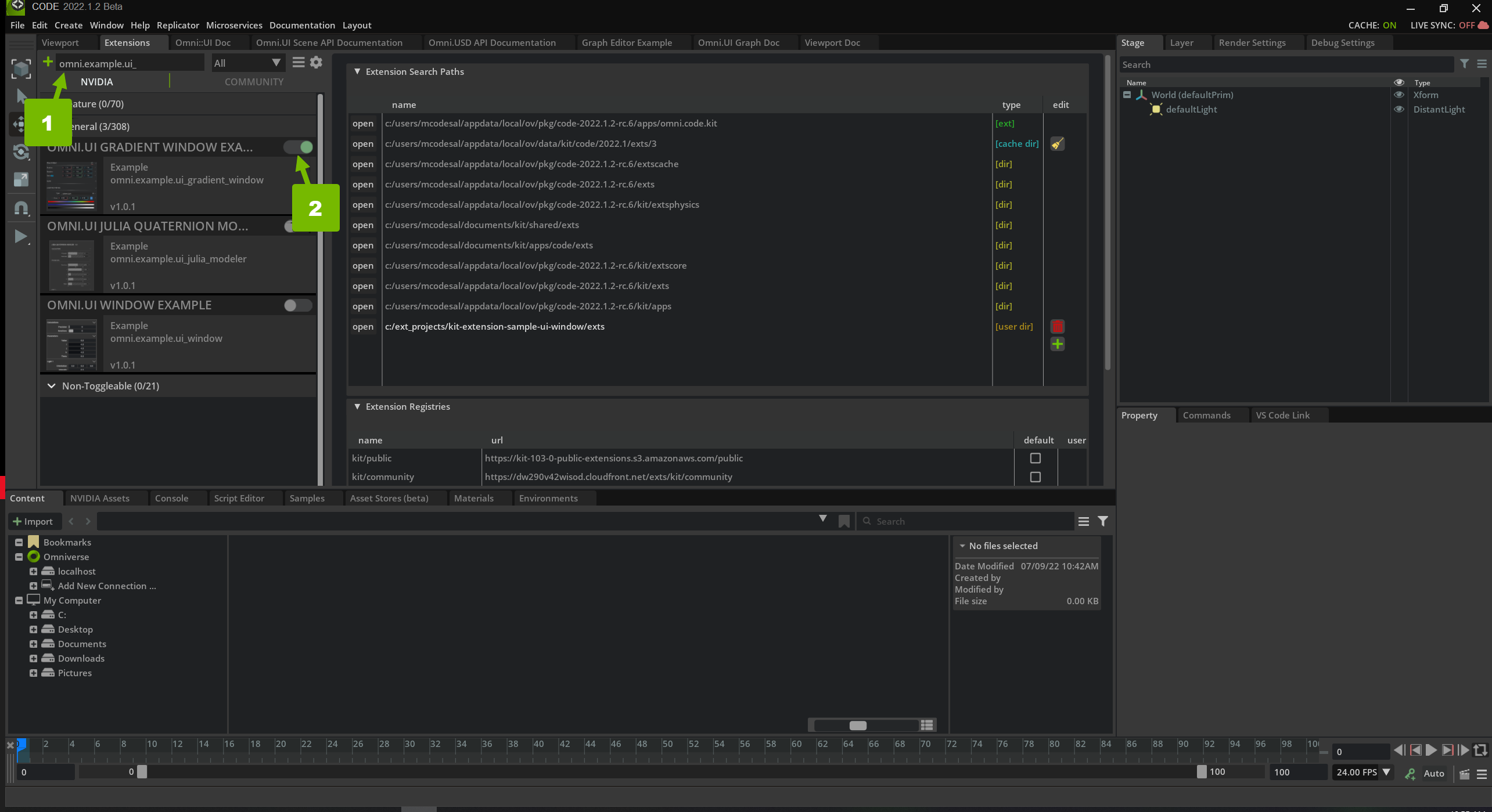After Enabling the extension the following window will appear: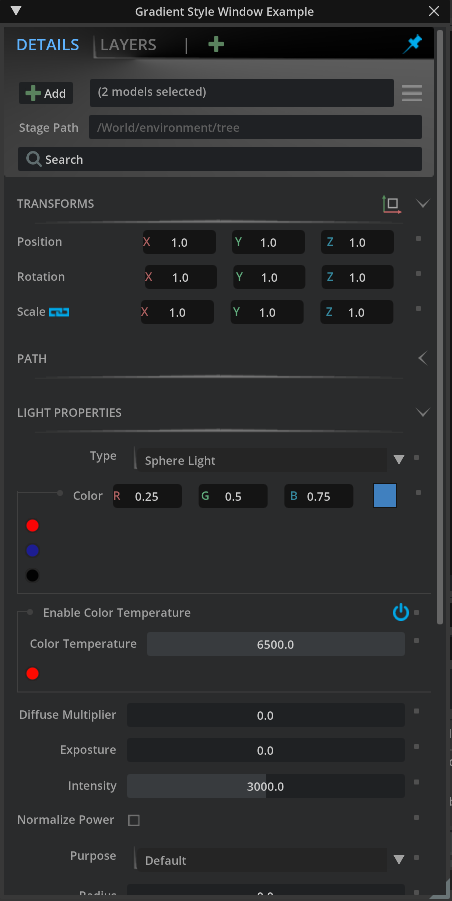Unlike the main repo, this extension is missing quite a few things that we will need to add, mainly the gradient. Moving forward we will go into detail on how to create the gradient style and apply it to our UI Window.

## Step 2: Familiarize Yourself with Interpolation

What is interpolation? Interpolation a way to find or estimate a point based on a range of discrete set of known data points. For our case we interpolate between colors to appropriately set the slider handle color.

Let’s say the start point is black and our end point is white. What is a color that is in between black and white? Gray is what most would say. Using interpolation we can get more than just gray. Here is a picture representation of what it looks like to interpolate between black and white:We can also use blue instead of black. It would then look something like this:Interpolation can also be used with a spectrum of colors:## Step 3: Set up the Gradients

Hexadecimal (Hex) is a base 16 numbering system where `0-9` represents their base 10 counterparts and `A-F` represent the base 10 values `10-15`.

A Hex color is written as `#RRGGBB` where `RR` is red, `GG` is green and `BB` is blue. The hex values have the range `00` - `ff` which is equivalent to `0` - `255` in base 10. So to write the hex value to a color for red it would be: `#ff0000`. This is equivalent to saying `R=255, G=0, B=0`.

To flesh out the `hex_to_color` function we will use bit shift operations to convert the hex value to color.

### Step 3.1: Navigate to `style.py`

Open the project in VS Code and open the `style.py` file inside of `omni.example.ui_gradient_window\omni\example\ui_gradient_window`

Tip

Remember to open up any extension in VS Code by browsing to that extension in the Extension tab, then select the extension and click on the VS Code logo.

Locate the function `hex_to_color` towards the bottom of the file. There will be other functions that are not yet filled out:

```def hex_to_color(hex: int) -> tuple:
pass

def generate_byte_data(colors):
pass

def _interpolate_color(hex_min: int, hex_max: int, intep):
pass

pass

pass
```

Currently we have the `pass` statement in each of the functions because each function needs at least one statement to run.

Warning

Removing the pass in these functions without adding any code will break other features of this extension!

### Step 3.2: Add Red to `hex_to_color`

Replace `pass` with `red = hex & 255`:

```def hex_to_color(hex: int) -> tuple:
# convert Value from int
red = hex & 255
```

Warning

Don’t save yet! We must return a tuple before our function will run.

### Step 3.3: Add Green to `hex_to_color`

Underneath where we declared `red`, add the following line `green = (hex >> 8) & 255`:

```def hex_to_color(hex: int) -> tuple:
# convert Value from int
red = hex & 255
green = (hex >> 8) & 255
```

Note

255 in binary is 0b11111111 (8 set bits)

### Step 3.4: Add the remaining colors to `hex_to_color`

Try to fill out the rest of the following code for `blue` and `alpha`:

```def hex_to_color(hex: int) -> tuple:
# convert Value from int
red = hex & 255
green = (hex >> 8) & 255
rgba_values = [red, green, blue, alpha]
return rgba_values
```
```def hex_to_color(hex: int) -> tuple:
# convert Value from int
red = hex & 255
green = (hex >> 8) & 255
blue = (hex >> 16) & 255
alpha = (hex >> 24) & 255
rgba_values = [red, green, blue, alpha]
return rgba_values
```

## Step 4: Create `generate_byte_data`

We will now be filling out the function `generate_byte_data`. This function will take our colors and generate byte data that we can use to make an image using `ImageWithProvider`. Here is the function we will be editing:

```def generate_byte_data(colors):
pass
```

### Step 4.1: Create an Array for Color Values

Replace `pass` with `data = []`. This will contain the color values:

```def generate_byte_data(colors):
data = []
```

### Step 4.2: Loop Through the Colors

Next we will loop through all provided colors in hex form to color form and add it to `data`. This will use `hex_to_color` created previously:

```def generate_byte_data(colors):
data = []
for color in colors:
data += hex_to_color(color)
```

### Step 4.3: Loop Through the Colors

Use ByteImageProvider to set the sequence as byte data that will be used later to generate the image:

```def generate_byte_data(colors):
data = []
for color in colors:
data += hex_to_color(color)

_byte_provider = ui.ByteImageProvider()
_byte_provider.set_bytes_data(data [len(colors), 1])
return _byte_provider
```

## Step 5: Build the Image

Now that we have our data we can use it to create our image.

### Step 5.1: Locate `build_gradient_image()`

In `style.py`, navigate to `build_gradient_image()`:

```def build_gradient_image(colors, height, style_name):
pass
```

### Step 5.2: Create Byte Sequence

Replace `pass` with `byte_provider = generate_byte_data(colors)`:

```def build_gradient_image(colors, height, style_name):
byte_provider = generate_byte_data(colors)
```

### Step 5.3: Transform Bytes into the Gradient Image

Use ImageWithProvider to transform our sequence of bytes to an image.

```def build_gradient_image(colors, height, style_name):
byte_provider = generate_byte_data(colors)
ui.ImageWithProvider(byte_provider,fill_policy=omni.ui.IwpFillPolicy.IWP_STRETCH, height=height, name=style_name)
return byte_provider
```

Save `style.py` and take a look at our window. It should look like the following: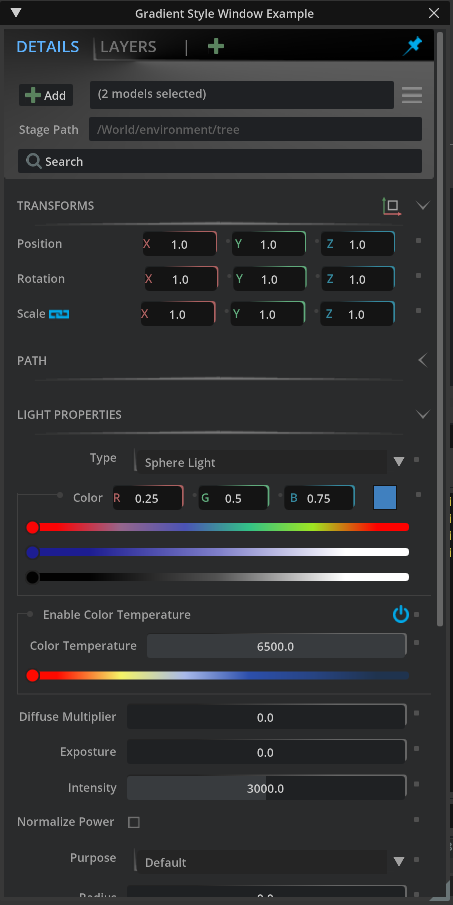Note

If the extension does not look like the following, close down Code and try to relaunch.

### Step 5.4: How are the Gradients Used?

Head over to `color_widget.py`, then scroll to around line 90:

```self.color_button_gradient_R = build_gradient_image([cl_attribute_dark, cl_attribute_red], 22, "button_background_gradient")
ui.Spacer(width=9)
with ui.VStack(width=6):
ui.Spacer(height=8)
ui.Circle(name="group_circle", width=4, height=4)
ui.Spacer(width=9)
with ui.VStack(width=6):
ui.Spacer(height=8)
ui.Circle(name="group_circle", width=4, height=4)
```

This corresponds to the widgets that look like this: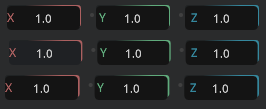### Step 5.5: Experiment - Change the red to pink

Go to `style.py`, locate the pre-defined constants, change `cl_attribute_red`’s value to `cl("#fc03be")`

```# Pre-defined constants. It's possible to change them runtime.
fl_attr_hspacing = 10
fl_attr_spacing = 1
fl_group_spacing = 5

cl_attribute_dark = cl("#202324")
cl_attribute_red = cl("#fc03be") # previously was cl("#ac6060")
cl_attribute_green = cl("#60ab7c")
cl_attribute_blue = cl("#35889e")
cl_line = cl("#404040")
cl_text_blue = cl("#5eb3ff")
cl_text_gray = cl("#707070")
cl_text = cl("#a1a1a1")
cl_text_hovered = cl("#ffffff")
cl_field_text = cl("#5f5f5f")
cl_widget_background = cl("#1f2123")
cl_attribute_default = cl("#505050")
cl_attribute_changed = cl("#55a5e2")
cl_slider = cl("#383b3e")
cl_combobox_background = cl("#252525")
cl_main_background = cl("#2a2b2c")

cls_temperature_gradient = [cl("#fe0a00"), cl("#f4f467"), cl("#a8b9ea"), cl("#2c4fac"), cl("#274483"), cl("#1f334e")]
cls_color_gradient = [cl("#fa0405"), cl("#95668C"), cl("#4b53B4"), cl("#33C287"), cl("#9fE521"), cl("#ff0200")]
```

Tip

Storing colors inside of the `style.py` file will help with reusing those values for other widgets. The value only has to change in one location, inside of `style.py`, rather than everywhere that the hex value was hard coded.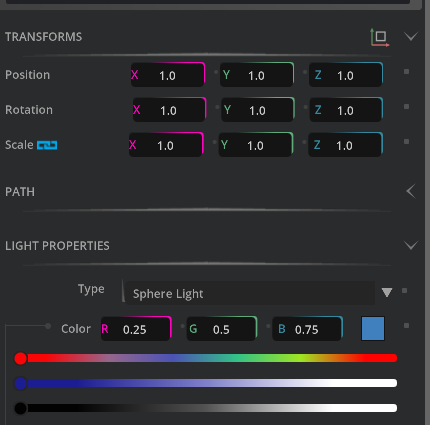The colors for the sliders can be changed the same way.

## Step 6: Get the Handle of the Slider to Show the Color as it’s Moved

Currently, the handle on the slider turns to black when interacting with it.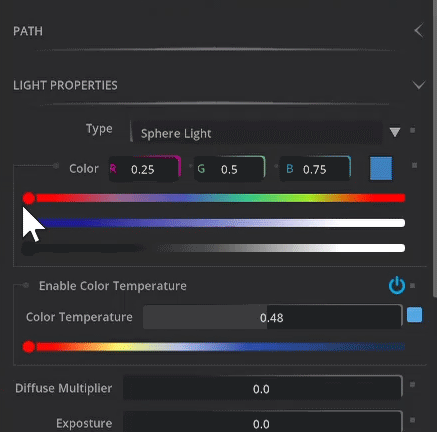This is because we need to let it know what color we are on. This can be a bit tricky since the sliders are simple images. However, using interpolation we can approximate the color we are on.

During this step we will be filling out `_interpolate_color` function inside of `style.py`.

```def _interpolate_color(hex_min: int, hex_max: int, intep):
pass
```

### Step 6.1: Set the color range

Define `max_color` and `min_color`. Then remove `pass`.

```def _interpolate_color(hex_min: int, hex_max: int, intep):
max_color = hex_to_color(hex_max)
min_color = hex_to_color(hex_min)
```

### Step 6.2: Calculate the color

```def _interpolate_color(hex_min: int, hex_max: int, intep):
max_color = hex_to_color(hex_max)
min_color = hex_to_color(hex_min)
color = [int((max - min) * intep) + min for max, min in zip(max_color, min_color)]
```

### Step 6.3: Return the interpolated color

```def _interpolate_color(hex_min: int, hex_max: int, intep):
max_color = hex_to_color(hex_max)
min_color = hex_to_color(hex_min)
color = [int((max - min) * intep) + min for max, min in zip(max_color, min_color)]
return (color << 8 * 3) + (color << 8 * 2) + (color << 8 * 1) + color
```

## Step 7: Getting the Gradient Color

Now that we can interpolate between two colors we can grab the color of the gradient in which the slider is on. To do this we will be using value which is the position of the slider along the gradient image, max being the maximum number the value can be, and a list of all the colors.

After calculating the step size between the colors that made up the gradient image, we can then grab the index to point to the appropriate color in our list of colors that our slider is closest to. From that we can interpolate between the first color reference in the list and the next color in the list based on the index.

### Step 7.1: Locate `get_gradient_color` function

```def get_gradient_color(value, max, colors):
pass
```

### Step 7.2: Declare `step_size` and `step`

```def get_gradient_color(value, max, colors):
step_size = len(colors) - 1
step = 1.0/float(step_size)
```

### Step 7.3: Declare `percentage` and `idx`

```def get_gradient_color(value, max, colors):
step_size = len(colors) - 1
step = 1.0/float(step_size)
percentage = value / float(max)

idx = (int) (percentage / step)
```

### Step 7.4: Check to see if our index is equal to our step size, to prevent an Index out of bounds exception

```def get_gradient_color(value, max, colors):
step_size = len(colors) - 1
step = 1.0/float(step_size)
percentage = value / float(max)

idx = (int) (percentage / step)
if idx == step_size:
color = colors[-1]
```

### Step 7.5: Else interpolate between the current index color and the next color in the list. Return the result afterwards.

```def get_gradient_color(value, max, colors):
step_size = len(colors) - 1
step = 1.0/float(step_size)
percentage = value / float(max)

idx = (int) (percentage / step)
if idx == step_size:
color = colors[-1]
else:
color = _interpolate_color(colors[idx], colors[idx+1], percentage)
return color
```

Save the file and head back into Omniverse to test out the slider.

Now when moving the slider it will update to the closest color within the color list.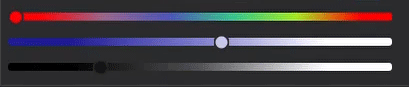## Conclusion

This was a tutorial about how to create gradient styles in the Window. Check out the complete code in the main branch to see how other styles were created. To learn more about how to create custom widgets check out the Julia Modeler example.

As a challenge, try to use the color that gets set by the slider to update something in the scene.